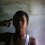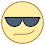### Program 218:One Time Pad Cipher using C

Program 218: To understand What is One Time Pad Cipher ?

```#include<stdio.h>
#include<string.h>
#include<ctype.h>
main()
{
//All the text which ever entered is converted to upper and without spaces
int i,j,len1,len2,numstr,numkey,numcipher;
char str,key,cipher;
printf("Enter a string text to encrypt\n");
gets(str);
for(i=0,j=0;i<strlen(str);i++)
{
if(str[i]!=' ')
{
str[j]=toupper(str[i]);
j++;
}
}
str[j]='\0';
//obtaining numerical plain text ex A-0,B-1,C-2
for(i=0;i<strlen(str);i++)
{
numstr[i]=str[i]-'A';
}
printf("Enter key string of random text\n");
gets(key);
for(i=0,j=0;i<strlen(key);i++)
{
if(key[i]!=' ')
{
key[j]=toupper(key[i]);
j++;
}
}
key[j]='\0';
//obtaining numerical one time pad(OTP) or key
for(i=0;i<strlen(key);i++)
{
numkey[i]=key[i]-'A';
}

for(i=0;i<strlen(str);i++)
{
numcipher[i]=numstr[i]+numkey[i];
}
//To loop the number within 25 i.e if addition of numstr and numkey is 27 then numcipher should be 1
for(i=0;i<strlen(str);i++)
{
if(numcipher[i]>25)
{
numcipher[i]=numcipher[i]-26;
}
}
printf("One Time Pad Cipher text is\n");
for(i=0;i<strlen(str);i++)
{
printf("%c",(numcipher[i]+'A'));
}
printf("\n");

}

```
Explanation:
Pre Explanation:

//Coming Soon...

Output:

1.there is code for decrypt?
I,m still newbie
thank u

1.2.thank you, it helps me a lot,
in OTP algorithm, can we encypt with 255 characters?

3.Dude, if you use "gets" as a function you kind of defeat the "perfect security" purpose, i know that this is probably not meant to be used professionally but using gets really exposes your code to a buffer overflow, it's a very bad practice in general.

1.https://stackoverflow.com/questions/1694036/why-is-the-gets-function-so-dangerous-that-it-should-not-be-used
here's a link on why you should forget gets existence

4.your program is very efficient.it have much help me.

1.how do I fix buffer overflow?

 Donate Buy me a coffee \$2.00 USD Buy me a burger \$5.00 USD Buy me a pizza \$10.00 USDCompiler Used by me C-Free 5.0(Recommended) Other Compilers Code Blocks(Recommended) Online Compilers Ideone(Remember to give input before executing online where ever necessary)